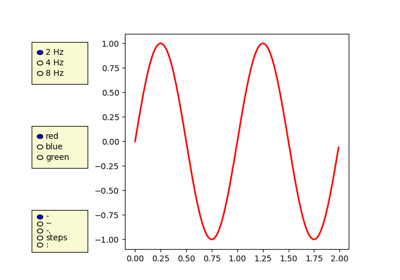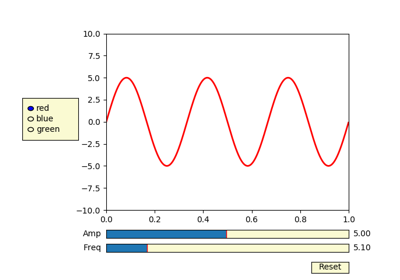# matplotlib.pyplot.axes¶

matplotlib.pyplot.axes(arg=None, **kwargs)[source]

Add an axes to the current figure and make it the current axes.

Parameters:
arg : None or 4-tuple or Axes

The exact behavior of this function depends on the type:

• None: A new full window axes is added using subplot(111, **kwargs)

• 4-tuple of floats rect = [left, bottom, width, height]. A new axes is added with dimensions rect in normalized (0, 1) units using add_axes on the current figure.

• Axes: This is equivalent to pyplot.sca. It sets the current axes to arg. Note: This implicitly changes the current figure to the parent of arg.

Note

The use of an Axes as an argument is deprecated and will be removed in v3.0. Please use pyplot.sca instead.

Returns:
axes : Axes

The created or activated axes.

Other Parameters:
**kwargs :

For allowed keyword arguments see pyplot.subplot and Figure.add_axes respectively. Some common keyword arguments are listed below:

kwarg Accepts Description
facecolor color the axes background color
frameon bool whether to display the frame
sharex otherax share x-axis with otherax
sharey otherax share y-axis with otherax
polar bool whether to use polar axes
aspect [str | num] ['equal', 'auto'] or a number. If a number, the ratio of y-unit/x-unit in screen-space. See also set_aspect.

Examples

Creating a new full window axes:

>>> plt.axes()


Creating a new axes with specified dimensions and some kwargs:

>>> plt.axes((left, bottom, width, height), facecolor='w')


## Examples using matplotlib.pyplot.axes¶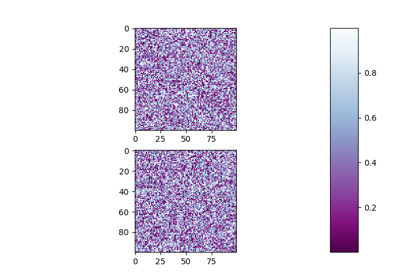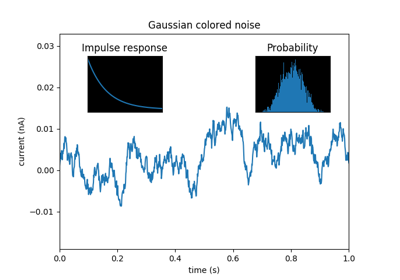Axes Demo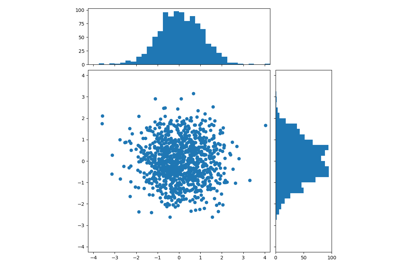Scatter Hist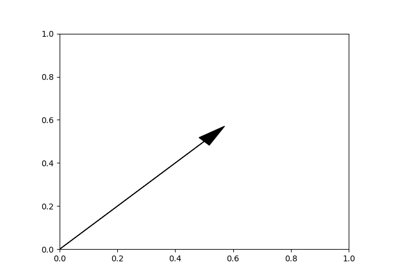Arrow Simple Demo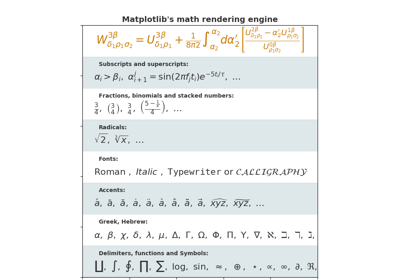Mathtext Examples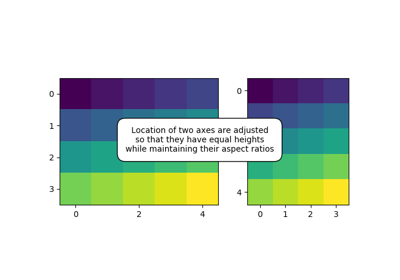Demo Axes Hbox Divider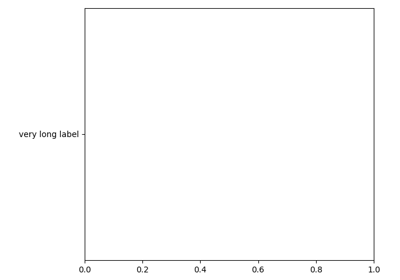Make Room For Ylabel Using Axesgrid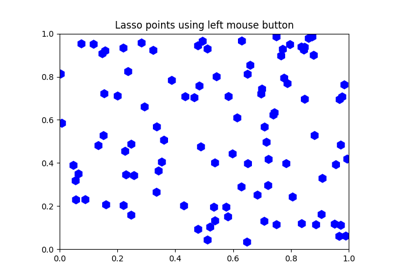Lasso Demo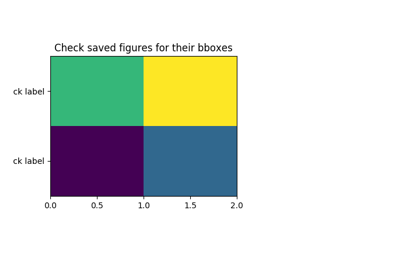Tight Bbox Test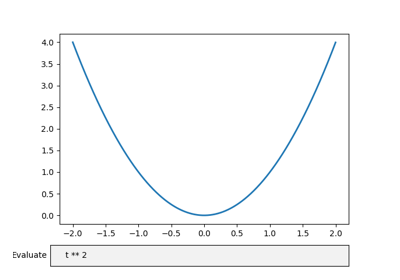Textbox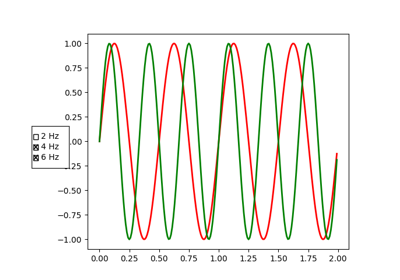Check Buttons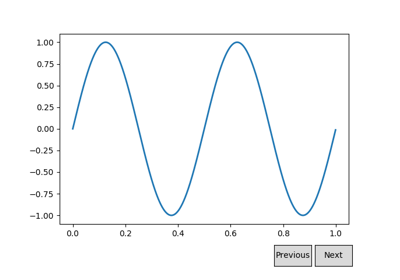Buttons